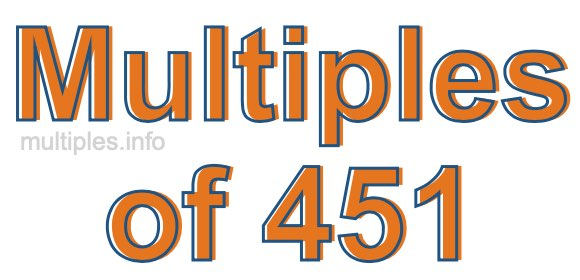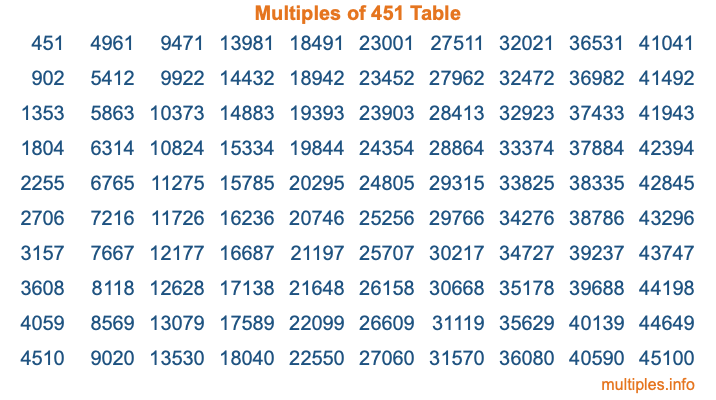Multiples of 451Welcome to the Multiples of 451 page. Here we will first teach you everything you will ever need to know about the multiples of 451, and then give you a study guide summary of everything we taught you to make sure you remember it all. Use this page to look up facts and learn information about the multiples of 451. This page will make you a multiples of four hundred fifty-one expert!

Definition of Multiples of 451
Multiples of 451 are all the numbers that when divided by 451 equal an integer. Each of the multiples of 451 are called a multiple. A multiple of 451 is created by multiplying 451 by an integer.

Therefore, to create a list of multiples of 451, you start with 1 multiplied by 451, then 2 multiplied by 451, then 3 multiplied by 451, and so on for as long as you want. Thus, the list of the first five multiples of 451 is 451, 902, 1353, 1804, and 2255. To see a larger list of multiples of 451, see the printable image of Multiples of 451 further down on this page. We also have a category where you can choose any nth multiple of 451.

Multiples of 451 Checker
The Multiples of 451 Checker below checks to see if any number of your choice is a multiple of 451. In other words, it checks to see if there is any number (integer) that when multiplied by 451 will equal your number. To do that, we divide your number by 451. If the the quotient is an integer, then your number is a multiple of 451.

Is  a multiple of 451?

Least Common Multiple of 451 and ...
A Least Common Multiple (LCM) is the lowest multiple that two or more numbers have in common. This is also called the smallest common multiple or lowest common multiple and is useful to know when you are adding our subtracting fractions. Enter one or more numbers below (451 is already entered) to find the LCM.

Check out our LCM Calculator if you need more details about the Least Common Multiple or if you need the LCM for different numbers for adding and subtraction fractions.

nth Multiple of 451
As we stated above, 451 is the first multiple of 451, 902 is the second multiple of 451, 1353 is the third multiple of 451, and so on. Enter a number below to find the nth multiple of 451.

th multiple of 451

Multiples of 451 vs Factors of 451
451 is a multiple of 451 and a factor of 451, but that is where the similarities end. All postive multiples of 451 are 451 or greater than 451. All positive factors of 451 are 451 or less than 451.

Below is the beginning list of multiples of 451 and the factors of 451 so you can compare:

Multiples of 451: 451, 902, 1353, 1804, 2255, etc.

Factors of 451: 1, 11, 41, 451

As you can see, the multiples of 451 are all the numbers that you can divide by 451 to get a whole number. The factors of 451, on the other hand, are all the whole numbers that you can multiply by another whole number to get 451.

It's also interesting to note that if a number (x) is a factor of 451, then 451 will also be a multiple of that number (x).

Multiples of 451 vs Divisors of 451
The divisors of 451 are all the integers that 451 can be divided by evenly. Below is a list of the divisors of 451.

Divisors of 451: 1, 11, 41, 451

The interesting thing to note here is that if you take any multiple of 451 and divide it by a divisor of 451, you will see that the quotient is an integer.

Multiples of 451 Table
Below is an image of the first 100 multiples of 451 in a table. The table is in chronological order, column by column. The first column has the first ten multiples of 451, the second column has the next ten multiples of 451, and so on.The Multiples of 451 Table is also referred to as the 451 Times Table or Times Table of 451. You are welcome to print out our table for your studies.

Negative Multiples of 451
Although not often discussed or needed in math, it is worth mentioning that you can make a list of negative multiples of 451 by multiplying 451 by -1, then by -2, then by -3, and so on, to get the following list of negative multiples of 451:

-451, -902, -1353, -1804, -2255, etc.

Multiples of 451 Summary
Below is a summary of important Multiples of 451 facts that we have discussed on this page. To retain the knowledge on this page, we recommend that you read through the summary and explain to yourself or a study partner why they hold true.

There are an infinite number of multiples of 451.

A multiple of 451 divided by 451 will equal a whole number.

451 divided by a factor of 451 equals a divisor of 451.

The nth multiple of 451 is n times 451.

The largest factor of 451 is equal to the first positive multiple of 451.

451 is a multiple of every factor of 451.

451 is a multiple of 451.

A multiple of 451 divided by a divisor of 451 equals an integer.

451 divided by a divisor of 451 equals a factor of 451.

Any integer times 451 will equal a multiple of 451.

Multiples of a Number
Here you can get the multiples of another number, all with the same attention to detail as we did for multiples of 451 on this page.

Multiples of
Multiples of 452
Did you find our page about multiples of four hundred fifty-one educational? Do you want more knowledge? Check out the multiples of the next number on our list!